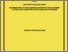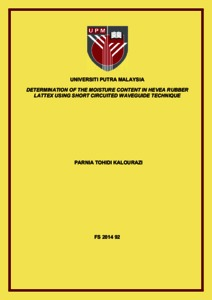# Determination of the moisture content in hevea rubber lattex using short circuited waveguide technique

## Citation

KaLourazi, Parnia Tohidi (2014) Determination of the moisture content in hevea rubber lattex using short circuited waveguide technique. Doctoral thesis, Universiti Putra Malaysia.

## Abstract

The thesis describes a short circuited waveguide technique for the determination of moisture content from reflection coefficient measurement of a waveguide loaded with Hevea rubber latex samples. The operating frequencies were from 8 GHz to 12 GHz. The theoretical part includes both the Finite Element Method and Short Circuit Line (SCL) theory to calculate the reflection coefficient of the sample placed in a waveguide. A computer program was written to implement the SCL calculation of the reflection coefficient due to the interaction between the electromagnetic waves and sample in the waveguide. Simulations using Finite Element Method was realized using the COMSOL software. The geometrical problem and the boundary conditions were first defined before performing the simulation of electromagnetic field distribution in the waveguide. The input permittivity of latex require for both SCL and FEM were obtained from measurements using Agilent Dielectric Probe Kit 85070B. Relationships between the dielectric constant, loss factor, moisture content and frequency have been established. The dielectric constant increases linearly with the measured frequency for all percentages of moisture content (% m.c). However the loss factor was inversely proportional to the operating frequencies. Samples with higher % m.c would give higher values of the dielectric constant and vice versa for the loss factor. The permittivity results were used in the calculation of the reflection coefficient. The FEM results were much closer to the measured values compared to the SCL theory for the whole frequency range between 8 GHz and 12GHz. Calibration equations to predict the % m.c from the measured reflection coefficient have been established. It was found the most accurate equation was based on the measurement of the magnitude of the reflection coefficient with accuracy within 3 % when compared to the actual mc obtained by the standard oven drying method.Preview
Text
FS 2014 92 IR.pdfView Item